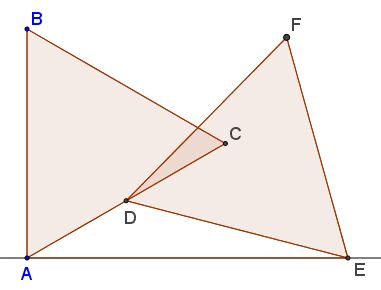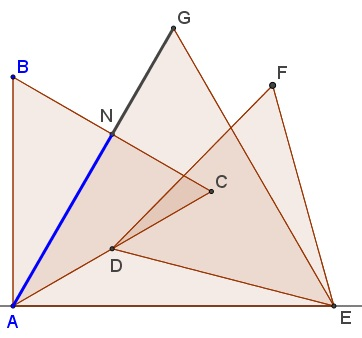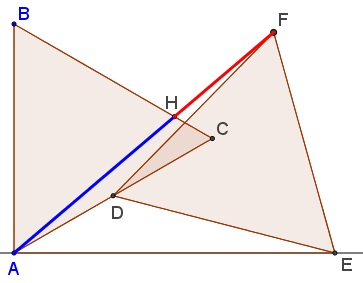# More of Golden Ratio in Equilateral Triangles

Elliot McGucken has messaged me his discovery of the Golden Ratio in a configuration of two equal equilateral triangles and the one obtained by the addition of a third triangle. All in all, three occurrences of the Golden Ratio. The proofs by Leo Giugiuc establish the fact for the first two occurrences. From the proof of the third occurrence we derive the presence of another one.

Here's the basic configuration ($\angle BAE=90^{\circ}\,$ $AD=CD\,$ and $AC=DE):$### The first occurrence of the Golden Ratio

Let $K\,$ be on $AE\,$ with $CK\perp AE.$Then $\displaystyle \frac{AK}{KE}=\varphi,\,$ the Golden Ratio.

Choose $A=(0,0),\,$ $B=(0,4),\,$ then $C=(2\sqrt{3},2),\,$ $D=(\sqrt{3},1),\,$ $K=(2\sqrt{3},0).\,$ $E=(x,0).\,$ Let's find $x:\,$ $\displaystyle \left(x-\sqrt{3}\right)^2+1=16,\,$ implying $x=2\sqrt{3}\varphi\,$ and, since $AK=2\sqrt{3},\,$ $\displaystyle EK=(\varphi-1)2\sqrt{3}=\frac{2\sqrt{3}}{\varphi}.\,$ It follows that $\displaystyle \frac{AK}{AE}=\varphi.$

### The second occurrence of the Golden Ratio

Let $AGE\,$ be another equilateral triangle, and $N\,$ the intersection of $BC\,$ and $AG.$Then $\displaystyle \frac{AN}{NG}=\varphi,\,$ the Golden Ratio.

In the above notations, $G=(\sqrt{3}\varphi,3\varphi).\,$ Also, $AG\,$ is defined by $y=x\sqrt{3}\,$ whereas $BC\,$ by $x+y\sqrt{3}=4\sqrt{3},\,$ so that $N=(\sqrt{3},3).\,$ In vector notations, $\overrightarrow{AN}=\sqrt{3}\vec{i}+3\vec{j}\,$ and $\displaystyle \overrightarrow{NG}=\frac{1}{\varphi}(\sqrt{3}\vec{i}+3\vec{j}).\,$ It follows that $\displaystyle \frac{AN}{NG}=\varphi,\,$ the Golden Ratio.

### The third occurrence of the Golden Ratio

Let $H\,$ be the intersection of $BC\,$ and $AF.$Then $\displaystyle \frac{AH}{HF}=\varphi,\,$ the Golden Ratio.

Define matrix $\mathbb{M}\,$ for the clockwise rotation through $60^{\circ}:$

$\displaystyle \mathbb{M}=\frac{1}{2}\begin{pmatrix}1&\sqrt{3}\\-\sqrt{3}&1\end{pmatrix}.$

Then

\displaystyle\begin{align} F&=E+\mathbb{M}\overrightarrow{DE}=\begin{pmatrix}2\sqrt{3}\varphi\\0\end{pmatrix}+\frac{1}{2}\begin{pmatrix}1&\sqrt{3}\\-\sqrt{3}&1\end{pmatrix}\begin{pmatrix}\sqrt{3}(1-2\varphi)\\1\end{pmatrix}\\ &=\begin{pmatrix}\sqrt{3}(1+\varphi)\\3\varphi-1\end{pmatrix}. \end{align}

Then $AF\,$ is described with $(3\varphi-1)x-\sqrt{3}(1+\varphi)y=0.\,$ Solving this together with the equation of $BC\,$ from above $(x+y\sqrt{3}=4\sqrt{3})\,$ gives $H=(\sqrt{3}\varphi,4-\varphi).\,$ Thus, following Leo Giugiuc's footsteps, we can write

\displaystyle\begin{align} \overrightarrow{AH}&=\sqrt{3}\varphi\vec{i}+(4-\varphi)\vec{j},\,\text{and}\\ \overrightarrow{HF}&=\sqrt{3}\vec{i}+(4\varphi-5)\vec{j}. \end{align}

So, it is easily seen that $\displaystyle \frac{AH}{HF}=\varphi,\,$ the Golden Ratio.

### The fourth occurrence of the Golden Ratio

Let $I\,$ be the intersection of $AB\,$ and $FG.$Then $\displaystyle \frac{AB}{BI}=\varphi,\,$ the Golden Ratio.

We shall simple show that $GF\parallel BC,\,$ and applying Thales's theorem, simultaneously recovering the previous occurrence of the Golden Ratio.

Thus $B=(0,4),\,$ $C=(2\sqrt{3},2)),\,$ $G=(\sqrt{3}\varphi,3\varphi)\,$ and $F=(\sqrt{3}(1+\varphi),3\varphi-1).\,$ Thus,

\displaystyle\begin{align} \overrightarrow{BC}&=2\sqrt{3}\vec{i}-2\vec{j},\,\text{and}\\ \overrightarrow{GF}&=\sqrt{3}\vec{i}-\vec{j}, \end{align}

indeed implying $GF\parallel BC.$### Golden Ratio